# ISEE Upper Level Math : Other Quadrilaterals

## Example Questions

### Example Question #1 : Other Quadrilaterals

Three of the interior angles of a quadrilateral measure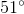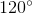, and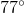. What is the measure of the fourth interior angle?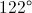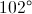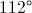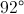Explanation:

The measures of the angles of a quadrilateral have sum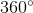. Ifis the measure of the unknown angle, then: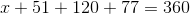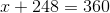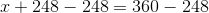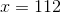The angle measures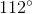.

### Example Question #2 : Other Quadrilaterals

The angles of a quadrilateral measure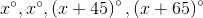. Evaluate.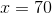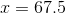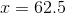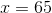Explanation:

The sum of the degree measures of the angles of a quadrilateral is 360, so we can set up and solve forin the equation: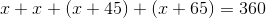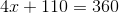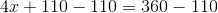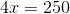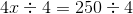### Example Question #3 : Other Quadrilaterals

The four angles of a quadrilateral have the following value: 79 degrees, 100 degrees, 50 degrees, anddegrees. What is the value of?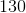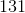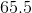Explanation:

Given that there are 360 degrees when all the angles of a quadrilateral are added toghether, this problem can be solved with the following equation: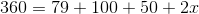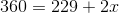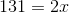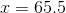### Example Question #4 : Other Quadrilaterals

In a quadrilateral, the angles have the following values: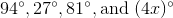What is the value of?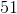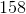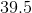Explanation:

Given that there are 360 degrees when the angles of a quadrilateral are added together, it follows that: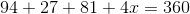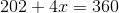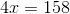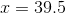### Example Question #231 : Plane Geometry

The perimeter of a quadrilateral is 86. The four sides measure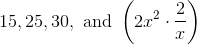.

What is the value of?Explanation:

Given that the perimeter is 86, the sides of the quadrilateral will all add up to this amount.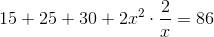The first step is the add together all the integers.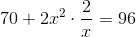Next, we subtract 70 from each side.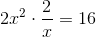We reduce the left side of the equation: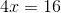Now, we divide each side by 4. This leaves: